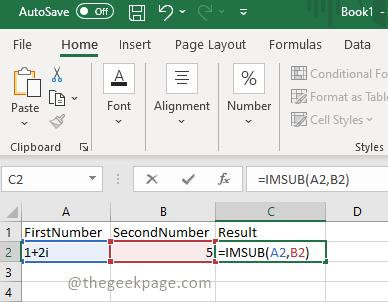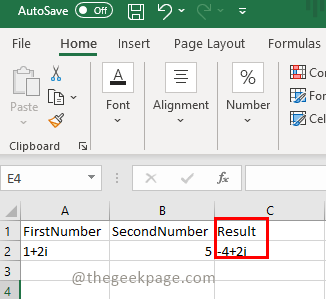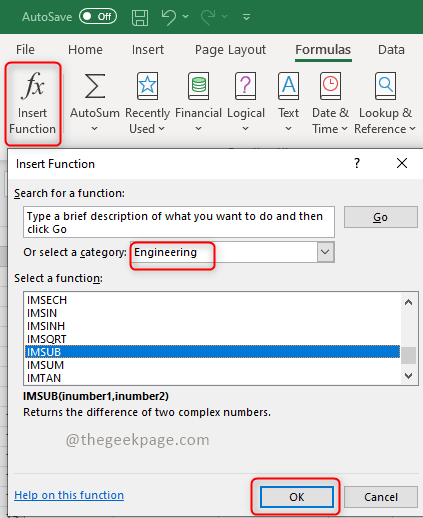# How to use IMSUB function in Microsoft Excel

Are you thinking about how can I work with Complex Numbers in excel? This article guides how to do it and it is very simple.  The Excel IMSUB function returns the difference between two complex numbers. One can choose any of the below methods to find the difference between complex numbers.

## Method 1: Calculate using IMSUB Formula

Step 1:  Open the excel and enter the complex numbers which need to be subtracted. The IMSUB formula goes like this =IMSUB(first number, second number)
Ex: Suppose we have two complex numbers in the columns FirstNumber and SecondNumber, type the above formula in the Result column to find the difference between them.Step 2: Once the formula is entered in the cell hit the Enter button to see the result.Step 3: If there is a table of numbers we can just drag the result tab and the formula will be automatically applied to all the rows.

## Method 2: Calculate the difference using Insert Function.

Step 1:  Click the Insert Function on the top left side in excel. Select Engineering from the category list and select IMSUB. Click OKStep 2: A Function Arguments dialog box will appear. Enter the cells to be calculated and click on OKStep 3: Hit the Enter button to see the result.That’s it! Isn’t it simple? Hope this article helped you. Do comment your thoughts below, Thank you !!!